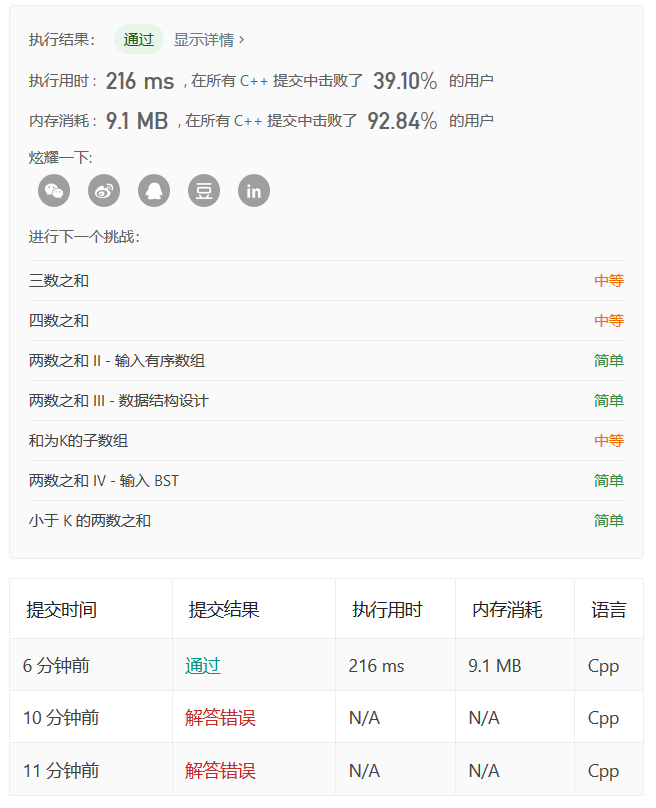C++代码实现

class Solution {
public:
vector<int> twoSum(vector<int>& nums, int target) {
vector<int> result;
for(int i = 0; i < nums.size() - 1; i++){
for(int j = i + 1; j < nums.size(); j++){
if(target == nums.at(i) + nums.at(j)){
result.push_back(i);
result.push_back(j);
break;
}
}
}
return result;
}
};

代码性能